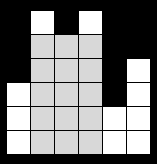### Problem Statement

A skyline is the outline formed by a group of buildings against the sky. A certain city has a beautiful skyline that's visible to everybody as they approach it by car. You have bought the rights to place an advertisement over it, and you would like to do so while preserving the shape of the city. The skyline is formed by n buildings, all with a width of 1 and each with a different height. You will place your ad on a rectangle of maximum area that is fully contained within the interior of the skyline.

To keep the input small, it will be codified in the following way. You will be given a int[] h. Use the following pseudo-code on h to generate an array R. The xth building has height R[x], which means its lower left corner is at (x, 0) and its upper right corner is at (x+1, R[x]). The total width of the skyline is n. All array indices are 0-based:

```input array: h
output array: R (of size n)
j := 0
m := size of h
for i := 0 to n-1
R[i] := h[j]
s := (j+1)%m
h[j] := ( ( h[j] ^ h[s] ) + 13 ) % 835454957
j := s
```

This code, along with the constraints, ensures that the height of each building is between 0 and 835454956, inclusive. In the above code, % is the modulo operator and ^ is the bitwise XOR binary operator. See the notes section for information on performing XOR in your language. Return the area of the rectangle on which you will place your ad.

### Definition

 Class: BuildingAdvertise Method: getMaxArea Parameters: int[], int Returns: long Method signature: long getMaxArea(int[] h, int n) (be sure your method is public)

### Notes

-The input is only coded for convenience. The intended solution does not rely on the way it is generated.
-If x and y are ints, (x^y) represents the bitwise XOR operation on them in C++, Java, C# and Python. In VB.Net (x BitXor y) does it.
-Note that the first elements of the input are exactly the corresponding elements of h.

### Constraints

-h will contain between 1 and 50 elements, inclusive.
-Each element of h will be between 0 and 835454956, inclusive.
-n will be between the number of elements in h and 100000, inclusive.

### Examples

0)

 `{3,6,5,6,2,4}` `6`
`Returns: 15`This is how the outline looks. The grayed area shows the optimal way to place the advertisement.
1)

 `{5,0,7,0,2,6,2}` `7`
`Returns: 7`
 Using building 2 entirely is the best choice.
2)

 `{1048589,2097165}` `100000`
`Returns: 104858900000`
 The resulting array is: {1048589, 2097165, 3145741, 1048589, 2097165, 3145741,..., 1048589, 2097165, 3145741, 1048589}.
3)

 `{1,7,2,5,3,1}` `6`
`Returns: 8`

#### Problem url:

http://www.topcoder.com/stat?c=problem_statement&pm=7473

#### Problem stats url:

http://www.topcoder.com/tc?module=ProblemDetail&rd=10661&pm=7473

soul-net

#### Testers:

PabloGilberto , brett1479 , Olexiy , Andrew_Lazarev

Search, Sorting